# Function Notation Worksheet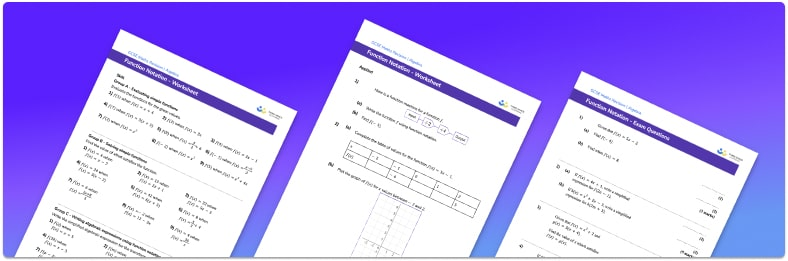• This field is for validation purposes and should be left unchanged.

You can unsubscribe at any time (each email we send will contain an easy way to unsubscribe). To find out more about how we use your data, see our privacy policy.

• Section 1 of the function notation worksheet contains 27 skills-based function notation questions, in 3 groups to support differentiation
• Section 2 contains 4 applied questions with a mix of worded problems and deeper problem solving questions
• Section 3 contains 4 foundation and higher level GCSE exam practice questions for function notation practice, including evaluating the function f for various values
• Answers and a mark scheme for all questions are provided
• Questions follow variation theory with plenty of opportunities for students to work independently at their own level
• All questions created by fully qualified expert secondary maths teachers
• Suitable for GCSE maths revision for AQA, OCR and Edexcel exam boards

### Functions at a glance

A function is a relationship between two variables.  There is a special notation for writing functions, such as f(x) which is read as the ‘function of x’. Each function within a question may be represented by a different letter such as f, g or h.

Evaluating functions requires us to substitute a value for x in the algebraic expression. Functions may be linear expressions or more complex polynomials or even algebraic fractions.

Functions can be used to produce pairs of coordinates (ordered pairs) and thus used to produce graphs of functions. Linear functions produce straight line graphs and quadratic functions produce curves.

Inverse functions have a special notation which looks like f-1(x) and composite functions have a notation which looks like fg(x) which is read as ‘f of g of x’.

We can also use our knowledge of function notation to produce situations where equations can be formed and solved.

Looking forward, students can then progress to additional algebra worksheets, for example a simplifying expressions worksheet or simultaneous equations worksheet.For more teaching and learning support on Algebra our GCSE maths lessons provide step by step support for all GCSE maths concepts.

## Related worksheets

Composite and Inverse Functions Worksheet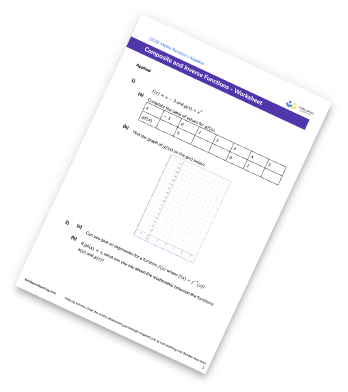Function Machines Worksheet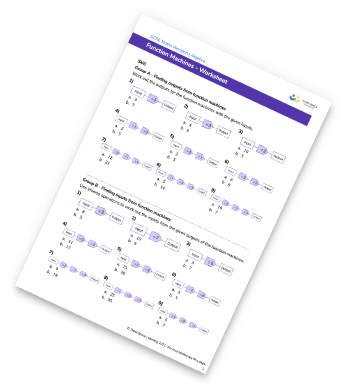Functions Worksheet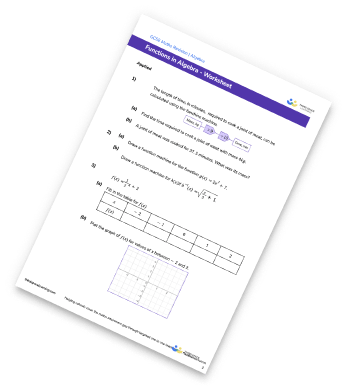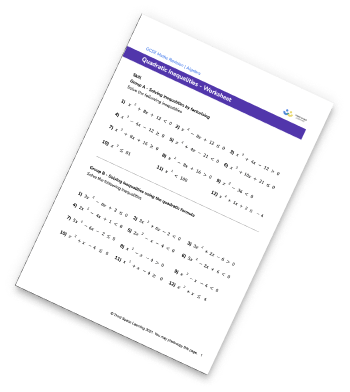## Do you have KS4 students who need more focused attention to succeed at GCSE?There will be students in your class who require individual attention to help them succeed in their maths GCSEs. In a class of 30, it’s not always easy to provide.

Help your students feel confident with exam-style questions and the strategies they’ll need to answer them correctly with our dedicated GCSE maths revision programme.

Lessons are selected to provide support where each student needs it most, and specially-trained GCSE maths tutors adapt the pitch and pace of each lesson. This ensures a personalised revision programme that raises grades and boosts confidence.

Find out more